# 代数入门――乘法

## 一个谜题

× 4 = 8

 x × 4 = 8

 4x = 8

 x = 2

## 怎样去解

• 想出移除什么可以得到 "x = 。。。。。。"
• 做相反的运算来移除它
• 每边都这么做

 需要移除"4" 要移除它，做相反的，在这里是除以 4： 每边都这么做： 这样。。。。。。 行了！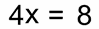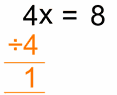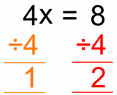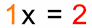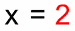## 为什么每边都要除以 4？

### "保持平衡"。。。。。。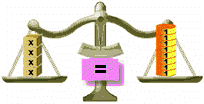平衡
 左边除以 4不平衡！
 右边也除以 4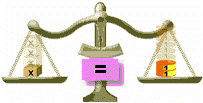恢复平衡了

### 记着。。。。。。

 要保持平衡，我们在 "=" 的一边做什么，在另一边也要做什么！

## 又一个谜题

 x / 3 = 5

 开始： x/3 = 5 我们的目标是像 "x = 。。。。。。"的答案，但有个 "除以 3" 堵在中间! 我们可以用 "乘以 3" 来抵消 "除以 3" (因为 3/3=1） 好了，我们每边乘以 3： x/3 ×3 = 5 ×3 用简单的算术 （3/3 = 1 和 5×3 = 15）： 1x = 15 结果是： x = 15 解了！ （快速检测：15/3 = 5）

## 较为复杂的例子

 x / 3 + 2 = 5

 开始： x/3 + 2 = 5 要移除 "加 2"，我们用 "减 2 " （因为 2-2=0） x/3 + 2 -2 = 5 -2 用简单的算术 （2-2 = 0 and 5-2 = 3）：： x/3 + 0 = 3 结果是： x/3 = 3

 开始： x/3 = 3 我们用 "乘以 3" 来抵消 "除以 3": x/3 ×3 = 3 ×3 用简单的算术 （3/3 = 1 和 3×3 = 9）： 1x = 9 结果是： x = 9 解了！ （快速检测：9/3 + 2 = 3+2 = 5）

## 熟能生巧

 开始： x/3 + 2 = 5 每边减 2： x/3 + 2 -2 = 5 -2 简化： x/3 = 3 每边乘以 3： x/3 ×3 = 3 ×3 简化： x = 9

 开始： x/3 + 2 = 5 减 2： x/3 = 3 乘以 3： x = 9

## 实例### 例子：小王淘了三盒巧克力。 邮费（还要邮费！）是￥9，总额（连邮费）是￥45。 每盒的价钱是多少？

3 乘 x 加 ￥9 是 ￥45：

3x + 9 = 45

 开始： 3x + 9 = 45 没变减 9： 3x + 9 − 9 = 45 − 9 简化： 3x = 36 除以 3： 3x /3 = 36 /3 简化： x = 12

 开始： 3x + 9 = 45 除以 3：: 3x/3 + 9/3 = 45/3 简化： x + 3 = 15 每边减以 3： x + 3 − 3 = 15 − 3 简化： x = 12